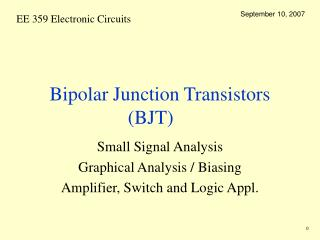Download PresentationBipolar Junction Transistors (BJT)

# Bipolar Junction Transistors (BJT)

Download Presentation## Bipolar Junction Transistors (BJT)

- - - - - - - - - - - - - - - - - - - - - - - - - - - E N D - - - - - - - - - - - - - - - - - - - - - - - - - - -
##### Presentation Transcript

1. September 10, 2007 Bipolar Junction Transistors (BJT) Small Signal Analysis Graphical Analysis / Biasing Amplifier, Switch and Logic Appl.

2. September 10, 2007 HW # 2 HW 2 due Friday 3.52, 3.72, 3.66 , 3.98

3. September 10, 2007 NPN Transistor Amplifier Example • NPN • Quiescent point

4. September 10, 2007 Small Signal Analysis

5. BJT as Amplifier September 10, 2007 BJT as a current-controlled current source (a current amplifier). BJT as a voltage-controlled current source ( a transconductance amplifier)

6. Small Signal September 10, 2007

7. September 10, 2007 Small Signal Analysis • Employ either hybrid-p model. • Using the first model • BJT as Amplifier Dependent Current Source C B VBE E

8. Signal Waveforms September 10, 2007

9. September 10, 2007 PNP Transistor Amplifier Example • Voltage Gain • Signal Waveforms • Capacitor couples input signal vi to emitter • DC bias with V+ &V-

10. September 10, 2007 DC Analysis • Find operating pt. Q • Let b=100 and a=0.99 • The transistor is active • Max. signal swing depends on bias voltage

11. September 10, 2007 Small Signal Analysis • Replace BJT with T equivalent ckt. • Why? Base is gnded. More convenient than hybrid p • a= 0.99 re=25mV/0.93mA= 27 W

12. September 10, 2007 Small Signal Equiv Ckt • VO/Vi =0.99x5k/27=183 • Allowable signal magnitude? • But veb = vi For small signal limit to 10mV. Then, vc=1.833V

13. September 10, 2007 Graphical Analysis • Find DC bias point • Set vi=0 and draw load line to determine dc bias point IB (similar to diode ckts)

14. September 10, 2007 Graphical Construction • Load line has a slope of –1/RB • iB vs vBE from forward biased diode eqns Graphical construction for the determination of the dc base current

15. Collector Current September 10, 2007 Graphical construction for determining the dc collector current IC and the collector-to-emmiter voltage

16. September 10, 2007 Small Signal Graphical Analysis • Signal is superimposed on DC voltage VBB • Corresponding to each instantaneous value of VBB + vi(t) draw a load line • Intersection of the iB -vBE curve with the load lines • Amplitude vi(t) small so ib linear

17. September 10, 2007 Collector Currrent • Corresponding to each instantaneous value of VCE + vce(t) operating point will be on the load line • Amplitude vi(t) small so ic linear

18. September 10, 2007 Bias Point vs Signal Swing • Bias-point location limits allowable signal swing • Load-line A results in bias point QA with a corresponding VCE which is too close to VCC and thus limits the positive swing of vCE. • At the other extreme, load-line B results in an operating point too close to the saturation region, thus limiting the negative swing of vCE.

19. Basic Single Stage Amplifiers September 10, 2007 Common-emitter amplifier with a resistance Re in the emitter. (a) Circuit. (b) Equivalent circuit with the BJT replaced with its T model (c) The circuit in (b) with ro eliminated.

20. Common Base Amp September 10, 2007 The common-base amplifier. (a) Circuit. (b) Equivalent circuit obtained by replacing the BJT with its T model.

21. Common Collector September 10, 2007 The common-collector or emitter-follower amplifier. (a) Circuit. (b) Equivalent circuit obtained by replacing the BJT with its T model.

22. September 10, 2007 (c) The circuit redrawn to show that ro is in parallel with RL. (d) Circuit for determining Ro.

23. General Large Signal Model September 10, 2007 An npn resistor and its Ebers-Moll (EM) model. The scale or saturation currents of diodes DE (EBJ) and DC (CBJ) are indicated in parentheses.

24. September 10, 2007 The transport model of the npn BJT. This model is exactly equivalent to the Ebers-Moll model Saturation currents of the diodes in parentheses

25. BJT Digital Logic September 10, 2007 Basic BJT digital logic inverter.

26. September 10, 2007 • voltage transfer characteristic of the inverter circuit • RB = 10 k, RC = 1 k,  = 50, and VCC = 5V.

27. Saturation Region September 10, 2007 The minority-carrier concentration in the base of a saturated transistor is represented by line (c). (b) The minority-carrier charge stored in the base can de divided into two components: That in blue produces the gradient that gives rise to the diffusion current across the base, and that in gray results in driving the transistor deeper into saturation.

28. September 10, 2007 The ic-vcb or common-base characteristics of an npn transistor. Note that in the active region there is a slight dependence of iC on the value of vCB. The result is a finite output resistance that decreases as the current level in the device is increased.

29. Common Base Characteristic September 10, 2007 The hybrid-p model, including the resistance r, which models the effect of vc on ib.

30. Common-emitter characteristics. September 10, 2007

31. Common Emitter in Saturation Region September 10, 2007